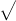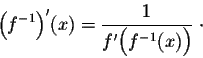# Help with inverse of derivative function

• Hazy001

#### Hazy001

Member warned about not using the homework template
f(x) =3x^3 + 3x^2+ 2x + 1
,a = 3

formal isHomework is due tonight and this is the only problem i can't solve

3=3x^3 + 3x^2 + 2x + 1
, solve for xThe find the derivative of y=3x^3 + 3x^2 + 2x + 1
, then plug x into that and put it under 1.

f(x) =3x^3 + 3x^2+ 2x + 1
,a = 3
What does a represent? I infer from your work below that you are taking it to mean the point (x0, a) on the graph of f.
Hazy001 said:
formal isHomework is due tonight and this is the only problem i can't solve

3=3x^3 + 3x^2 + 2x + 1
, solve for xThe find the derivative of y=3x^3 + 3x^2 + 2x + 1
, then plug x into that and put it under 1.